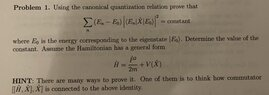# Quantum Mechanics problem: Determine the value of the constant

Homework Statement:
The problem states work for word.

Using canonical quantization relation, prove that
sum operator ((E_n -E_0)) |< E_n | X | E_0 >|^2) = constant

Where E_0 is the energy corresponding to the eigenstate | E_0 >. Determine the value of the constant. Assume the hamiltonian had a general form H = P/2m +V(X)

Hint: One way to proof this is to think how [H, X], X] is connected to the obove identity.
Relevant Equations:
all equations i have are in the statement.
I have no idea where to start with this problem. I am interested in any hints, or ways to proof this. But i would especially like to know how the commutator is connected to the identity.

#### Attachments

•385A8420-90F2-4204-8439-15C0224B4160.jpeg
25.8 KB · Views: 21

Mentor
Please, everyone, be respectful of poster asking for a hint about one specific aspect of this problem.

•topsquark, Lord Jestocost and berkeman
Gold Member
MHB
Homework Statement:: The problem states work for word.

Using canonical quantization relation, prove that
sum operator ((E_n -E_0)) |< E_n | X | E_0 >|^2) = constant

Where E_0 is the energy corresponding to the eigenstate | E_0 >. Determine the value of the constant. Assume the hamiltonian had a general form H = P/2m +V(X)

Hint: One way to proof this is to think how [H, X], X] is connected to the obove identity.
Relevant Equations:: all equations i have are in the statement.

I have no idea where to start with this problem. I am interested in any hints, or ways to proof this. But i would especially like to know how the commutator is connected to the identity.
What is ##< E_0 \mid [H,X],X]] \mid E_0 >##?

-Dan

•vanhees71
•